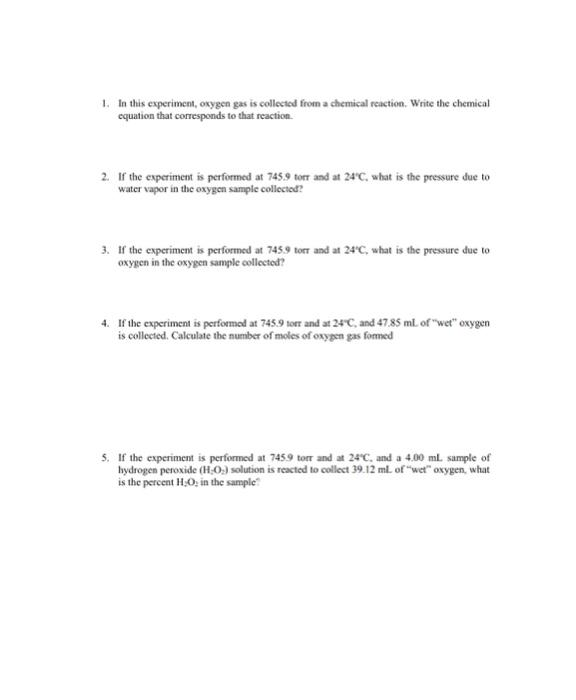Home / Expert Answers / Chemistry / 1-in-this-experiment-oxygen-gas-is-collected-from-a-chemical-reaction-write-the-chemical-equatio-pa413

# (Solved): 1. In this experiment, oxygen gas is collected from a chemical reaction. Write the chemical equatio ...1. In this experiment, oxygen gas is collected from a chemical reaction. Write the chemical equation that corresponds to that reaction. 2. If the experiment is performed at 745.9 torr and at , what is the pressure due to water vapor in the oxygen sample collected? 3. If the experiment is performed at 745.9 torr and at , what is the pressure due to oxygen in the oxygen sample collected? 4. If the experiment is performod at 745.9 torr and at , and of "wet" oxygen is collected. Calculate the number of moles of oxypen gas formed 5. If the experiment is performed at 745.9 torr and at , and a sample of bydrogen peroxide solution is reacted to collect . of "wet" oxygen, what is the percent in the sample?

We have an Answer from Expert